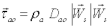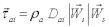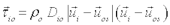# Surface Stress

Surface Stresses

• Calculate daily surface wind stress over the open ocean as:• The wind stress over the sea ice is formulated identically as:• Calculate surface stress over the sea-ice covered ocean as:• The ocean current stress at the base of the sea-ice cover is precisely the negative of the above.
• Note that a turning angle is not applied to the ocean surface currents.
• Dao, Dai and Dio are air-ocean, air-ice and ice-ocean drag coefficients:

Dao=(1.10 + 0.04 Ws) x  10-3Dai=(1.10 + 0.04 Ws) x  10-3Dio=5.50 x  10-3;

• Dao, Dai and Dio are also discussed in section “Forcing Data Notation” and in “Model details “ for different AOMIP models